/

### Extreme Events – Specimen Question A.2.2(b) – Answer/Hints

Q. Using a first order autoregressive model, de-smooth the observed returns for Index B to derive a return series that you think may provide a better measure of the underlying behaviour of the relevant asset category.

The approach for de-smoothing (or ‘de-correlating’) such returns suggested in the book Extreme Events involves assuming that there is some underlying ‘true’ return series,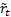, and that the observed series,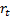, derives from it via a first order autoregressive model,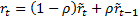. We will assume that the autoregressive model actually applies to the logged returns, which are given in ExtremeEventsQuestionsAndAnswers2_1a.

If we assume that the correspondingare independent, identically distributed (normal) random variables with standard deviation(and mean 0 given that we have standardised the data) then the (expected) variance of the series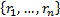, will be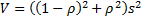and the (expected) covariance of the series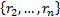with the series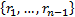will be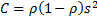.

One way of proceeding would be to estimate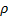as the solution to the equation: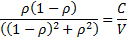More precisely, since the above does not differentiate betweenand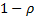we would probably choose theclosest to zero (and, ideally, we would expect it to be positive and smaller than 0.5, which also requires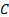to be positive, as a value ofoutside this range would be implausible).

In this instance:

 Statistic (using logged returns) Value (Sample*) Variance of(=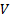) 0.008575828 (Sample*) Covariance ofwith(=) 0.002896449 Ratio (=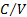) 0.337746 Estimated0.279955

* Ideally, the Variance and Covariance should both include the same small sample size adjustment, i.e. both be “sample” or both be “population” estimates. The Microsoft Excel functions, VAR, VARP and COVAR, are somewhat confusing in this respect, since COVAR is calculated using a multiplier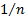, and is therefore properly a “population” statistic and consistent with VARP, whilst VAR is calculated using a multiplier of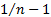, and so is a “sample” statistic. The Nematrian website’s web functions are clearer as it provides both a MnCovariance function and a MnPopulationCovariance function.

Ignoring small sample size adjustments, estimates ofcan be then be found using: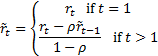However, if we do this in practice we find that there are second order effects that mean that estimatingas above still leaves some residual autocorrelation:

 Period(logged returns of B)(first pass) 1 0.0487902 0.0487902 2 -0.1743534 -0.2611119 3 -0.1255632 -0.0728617 4 -0.0304592 -0.0139731 5 -0.0976128 -0.1301320 6 -0.0010005 0.0492060 7 -0.0801260 -0.1304104 8 0.0276152 0.0890558 9 0.1231022 0.1363395 10 0.0148886 -0.0323317 11 -0.1109316 -0.1414913 12 0.1106465 0.2086780 13 0.1646666 0.1475549 14 0.0925792 0.0712046 15 0.0797350 0.0830516 16 -0.0650720 -0.1226626 17 -0.0812101 -0.0650933 18 -0.0232686 -0.0070071 19 0.0525925 0.0757649 20 0.0276152 0.0088945 Variance (=) 0.0085758 0.0138041 Covariance (=) 0.0028964 0.0007054 Ratio (=) 0.3377457 0.0511003 Estimated0.2799546

Better, therefore, is to use a root search algorithm in which we explicitly search for the(ideally between 0 and 0.5) for whichhas zero autocorrelation. This can be done using the Nematrian MnDesmooth_AR1 or MnDesmooth_AR1_rho functions (the former returns the desmoothed series, the latter returns the value offor whichhas zero autocorrelation). Given the form of the problem given here, these provide the following de-smoothed series (with rho equal to 0.31094682):

 Period(logged returns of B)(de-smoothed) 1 0.0487902 0.0487902 2 -0.1743534 -0.2750507 3 -0.1255632 -0.05810445 4 -0.0304592 -0.01798381 5 -0.0976128 -0.13354672 6 -0.0010005 0.05881321 7 -0.0801260 -0.14282465 8 0.0276152 0.10452905 9 0.1231022 0.13148365 10 0.0148886 -0.03772687 11 -0.1109316 -0.14396646 12 0.1106465 0.22554488 13 0.1646666 0.13719425 14 0.0925792 0.07244591 15 0.0797350 0.08302433 16 -0.0650720 -0.13190296 17 -0.0812101 -0.05833410 18 -0.0232686 -0.00744471 19 0.0525925 0.07968530 20 0.0276152 0.00411769 Variance (=) 0.0085758 0.01487681 Covariance (=) 0.0028964 0 Ratio (=) 0.3377457 0

Note:

(a)          In general de-smoothing increases the variance of the return series being analysed. Here it has gone from 0.0926 to 0.1219.

(b)          Whilst the problem would usually be stated as shown, it perhaps makes more sense not to make the arbitrary implicit assumption that the smoothing is around a mean of zero, but around some mean (that is, for example, estimated from the data), i.e. as if the model was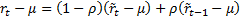.

(c)           We might not necessarily want to give equal weight to each observation. This is possible using the Nematrian MnWeightedDesmooth_AR1 and MnWeightedDesmooth_AR1_rho functions.